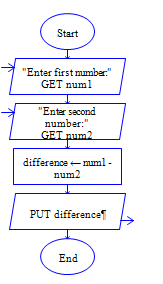# C Program to subtraction of two numbers

Get two integer numbers, subtract both the integers and display the difference

Sample Input 1:

6 5

Sample Output 1:

1

Sample Input 2:

65 4

Sample Output 2:

61

#### Flow Chart Design#### Program or Solution

``` //Program to subraction of two numbers #include<stdio.h> int main() { int num1,num2,diff; printf("Enter two numbers:"); scanf("%d %d",&num1,&num2); diff=num1-num2; printf("\nDifference is : %d",diff); return 0; } ```

#### Program Explanation

Get two integers a and b (using scanf statement) Subract b from a, then store the difference in c (c=a-b) print the value of c (using printf statement)